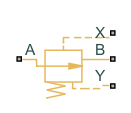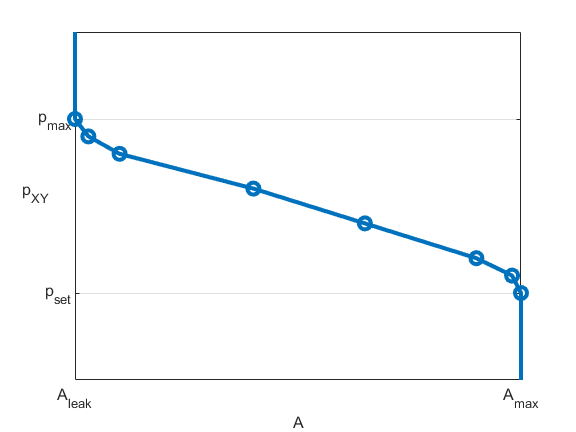# Pressure Compensator Valve (TL)

Pressure compensator valve in thermal liquid network

Since R2023a

•Libraries:
Simscape / Fluids / Thermal Liquid / Valves & Orifices / Pressure Control Valves

## Description

The Pressure Compensator Valve (TL) block represents a pressure compensator in a thermal liquid network, such as a pressure relief valve or pressure reducing valve. Use this block to maintain the pressure at the valve based on signals from another part of the system.

The pressure differential between ports X and Y is the control pressure, Pcontrol. When this value meets or exceeds the set pressure, the valve area opens or closes depending on the Valve specification parameter. The pressure regulation range begins at the set pressure, Pset.

### Pressure Control

The block regulates pressure when Pcontrol exceeds Pset and continues to regulate the pressure until Pmax, the sum of Pset and the pressure regulation range. The Set pressure differential parameter defines the constant regulation range.

### Conservation of Mass

The block conserves mass such that

`${\stackrel{˙}{m}}_{A}+{\stackrel{˙}{m}}_{B}=0.$`

The block calculates the mass flow rate through the valve as

`$\stackrel{˙}{m}=\frac{{C}_{d}{A}_{valve}\sqrt{2\overline{\rho }}}{\sqrt{P{R}_{loss}\left(1-{\left(\frac{{A}_{valve}}{{A}_{port}}\right)}^{2}\right)}}\frac{\Delta p}{{\left[\Delta {p}^{2}+\Delta {p}_{crit}^{2}\right]}^{1/4}},$`

where:

• Cd is the value of the Discharge coefficient parameter.

• Avalve is the instantaneous valve open area.

• Aport is the value of the Cross-sectional area at ports A and B parameter.

• $\overline{\rho }$ is the average fluid density.

• Δp is the valve pressure difference pApB.

The critical pressure difference, Δpcrit, is the pressure differential specified by the Critical Reynolds number parameter, Recrit. This parameter represents the flow regime transition point between laminar and turbulent flow. The block finds the critical pressure difference as

`$\Delta {p}_{crit}=\frac{\pi }{8{A}_{valve}\overline{\rho }}{\left(\frac{\mu {\mathrm{Re}}_{crit}}{{C}_{d}}\right)}^{2},$`

where μ is the dynamic viscosity of the thermal liquid.

The pressure loss, PRloss, describes the reduction of pressure in the valve due to a decrease in area. The block calculates the pressure loss as:

`$P{R}_{loss}=\frac{\sqrt{1-{\left(\frac{{A}_{valve}}{{A}_{port}}\right)}^{2}\left(1-{C}_{d}^{2}\right)}-{C}_{d}\frac{{A}_{valve}}{{A}_{port}}}{\sqrt{1-{\left(\frac{{A}_{valve}}{{A}_{port}}\right)}^{2}\left(1-{C}_{d}^{2}\right)}+{C}_{d}\frac{{A}_{valve}}{{A}_{port}}}.$`

The pressure recovery describes the positive pressure change in the valve due to an increase in area. When you clear the Pressure recovery check box, the block sets PRloss to 1.

The block calculates Avalve using the opening parameterization and the valve opening dynamics.

### Valve Opening Parameterization

When you set Opening parameterization to `Linear`, the valve area for normally open valves is

`${A}_{valve}=\stackrel{^}{p}\left({A}_{leak}-{A}_{max}\right)+{A}_{max},$`

where Aleak is the value of the Leakage Area parameter and Amax is the value of the Maximum opening area parameter. This figure shows how the block controls the opening area for a normally open valve using the linear parameterization.For normally closed valves, the block uses

`${A}_{valve}=\stackrel{^}{p}\left({A}_{max}-{A}_{leak}\right)+{A}_{leak}.$`

This figure show how the block controls the opening area for a normally closed valve using the linear parameterization.The normalized pressure, $\stackrel{^}{p}$, is

`$\stackrel{^}{p}=\frac{{p}_{control}-{p}_{set}}{{p}_{max}-{p}_{set}}.$`

When the valve is in a near-open or near-closed position in the linear parameterization, you can maintain numerical robustness in your simulation by adjusting the parameter. If the parameter is nonzero, the block smoothly saturates the control pressure between pset and pmax. For more information, see Numerical Smoothing.

When you set Opening parameterization to ```Tabulated data```, Aleak and Amax are the first and last parameters of the Opening area vector parameter, respectively. The block calculates the opening area as

`${A}_{valve}=tablelookup\left({p}_{control,TLU,ref},{A}_{TLU},{p}_{control},interpolation=linear,extrapolation=nearest\right),$`

where:

• pcontrol,TLU,ref = pTLU + poffset.

• pTLU is the Pressure differential vector parameter.

• poffset is an internal pressure offset that causes the valve to start closing when pcontrol,TLU,ref = pset.

• ATLU is the Opening area vector parameter.

This figure demonstrates how the block controls the opening area for a normally open valve using the tabulated data parameterization.This figure demonstrates how the block controls the opening area for a normally closed valve using the tabulated data parameterization.### Opening Dynamics

When you select Opening dynamics, the block introduces a control pressure lag where pcontrol becomes the dynamic control pressure, pdyn. The block calculates the instantaneous change in dynamic control pressure based on the Opening time constant parameter, τ:

`${\stackrel{˙}{p}}_{dyn}=\frac{{p}_{control}-{p}_{dyn}}{\tau }.$`

By default, the block clears the Opening dynamics check box. When Opening parameterization is `Linear`, a nonzero value for the Smoothing factor parameter provides additional numerical stability when the orifice is in near-closed or near-open position.

The block calculates the steady-state dynamics according to the Opening parameterization parameter based on the control pressure, pcontrol.

### Energy Balance

The energy conservation equation in the valve is

`${\varphi }_{A}+{\varphi }_{B}=0,$`

where:

• ϕA is the energy flow rate into the valve through port A.

• ϕB is the energy flow rate into the valve through port B.

## Ports

### Conserving

expand all

Thermal liquid conserving port associated with the A side of the valve.

Thermal liquid conserving port associated with the B side of the valve.

Thermal liquid conserving port associated with the pressure at point X, Px.

Thermal liquid conserving port associated with point Y, Py.

## Parameters

expand all

Normal operating condition of the pressure compensator valve. For a reducing valve, choose `Normally open` valve. For a relief valve, choose `Normally closed`.

Method used to parameterize the opening action of the valve.

Magnitude of the pressure differential that triggers pressure compensation.

Operational pressure range of the valve. The pressure regulation range defines the difference between the Set pressure differential parameter and the maximum valve operating pressure.

#### Dependencies

To enable this parameter, set Opening parameterization to `Linear`.

Cross-sectional area of the valve in the fully open position.

#### Dependencies

To enable this parameter, set Opening parameterization to `Linear`.

Sum of all gaps when the valve is in the fully closed position. An area smaller than this value saturates to the specified leakage area. This value contributes to numerical stability by maintaining continuity in the flow.

#### Dependencies

To enable this parameter, set Opening parameterization to `Linear`.

Vector of pressure differential values for the tabulated parameterization of the opening area. The vector elements must correspond one-to-one to the values in the Opening area vector parameter. The pressures must be in ascending order.

#### Dependencies

To enable this parameter, set Opening parameterization to `Tabulated data`.

Vector of opening area values for the tabulated parameterization of the opening area. The vector elements must correspond one-to-one to the values in the Pressure differential vector parameter. For normally open valves, list elements in descending order. For normally closed valves, list elements in ascending order.

The opening area vector must have the same number of elements as the Pressure differential vector parameter. The block uses linear interpolation between table data points.

#### Dependencies

To enable this parameter, set Opening parameterization to `Tabulated data`.

Cross-sectional area at the entry and exit ports A and B. The block uses this area in the pressure-flow rate equation that determines mass flow rate through the valve.

Correction factor that the block uses to account for discharge losses in theoretical flows.

Upper Reynolds number limit for laminar flow through the valve.

Continuous smoothing factor that the block uses to introduce a layer of gradual change to the flow response when the valve is in near-open or near-closed positions. Set this parameter to a nonzero value less than one to increase the stability of your simulation in these regimes.

#### Dependencies

To enable this parameter, set Opening parameterization to `Linear`.

Whether to account for pressure increase when fluid flows from a region of a smaller cross-sectional area to a region of larger cross-sectional area.

Whether to account for transient effects to the fluid system due to the valve opening. When you select this parameter, the block approximates the opening conditions by introducing a first-order lag in the flow response.

Time constant by which to compute the lag in the opening dynamics.

#### Dependencies

To enable this parameter, select Opening dynamics.

## Version History

Introduced in R2023a